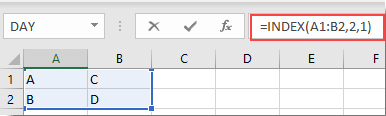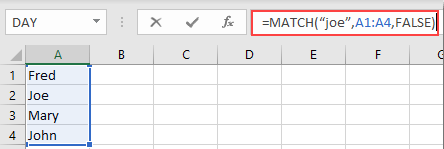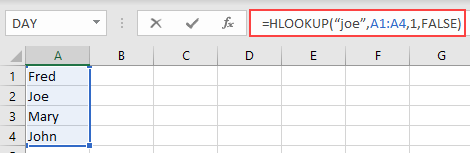Quiz

1. 1

## Suppose there is a small table with these values for the cells: A1 = A, A2 = B, B1 = C, B2=D. What does the formula =INDEX(A1:B2,2,1) return?2. 2